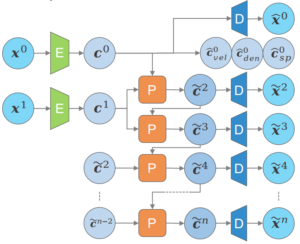Submitted to the Symposium on Computer Animation (SCA 2020).

Authors
Steffen Wiewel, Technical University of Munich
Byungsoo Kim, ETH Zurich
Vinicius C. Azevedo, ETH Zurich
Barbara Solenthaler, ETH Zurich
Nils Thuerey, Technical University of Munich

Abstract
We propose an end-to-end trained neural network architecture to robustly predict the complex dynamics of fluid flows with high temporal stability. We focus on single-phase smoke simulations in 2D and 3D based on the incompressible Navier-Stokes (NS) equations, which are relevant for a wide range of practical problems. To achieve stable predictions for long-term flow sequences, a convolutional neural network (CNN) is trained for spatial compression in combination with a temporal prediction network that consists of stacked Long Short-Term Memory (LSTM) layers. Our core contribution is a novel latent space subdivision (LSS) to separate the respective input quantities into individual parts of the encoded latent space domain. This allows to distinctively alter the encoded quantities without interfering with the remaining latent space values and hence maximizes external control. By selectively overwriting parts of the predicted latent space points, our proposed method is capable to robustly predict long-term sequences of complex physics problems. In addition, we highlight the benefits of a recurrent training on the latent space creation, which is performed by the spatial compression network.

Keywords
fluid simulation, neural networks, physics simulation, CNN, LSTM, machine learning, prediction, autoencoder, time series, deep sequence learningFurther Information
Computing the dynamics of fluids requires solving a set of complex equations over time. This process is computationally very expensive, especially when considering that the stability requirement poses a constraint on the maximal time step size that can be used in a simulation.
Due to the high computational resources, approaches for machine learning based physics simulations have recently been explored. One of the first approaches used Regression Forest as a regressor to forward the state of a fluid over time (Ladický et al., 2015). Handcrafted features have been used, representing the individual terms of the Navier-Stokes equations. These context-based integral features can be evaluated in constant time and robustly forward the state of the system over time. In contrast, using neural networks for the time prediction has the advantage that no features have to be defined manually, and hence these methods have recently gained increased attention. In graphics, the presented neural prediction methods (Wiewel et al., 2019; Kim et al., 2019; Morton et al., 2018) use a two-step approach, where first the physics fields are translated into a compressed representation, i.e., the latent space. Then, a second network is used to predict the state of the system over time in the latent space. The two networks are trained individually, which is an intuitive approach as spatial and temporal representations can be separated by design. In practice, the first network (i.e., the autoencoder) introduces small errors in the encoding and decoding in each time step. In combination with a temporal prediction network these errors accumulate over time, introducing drifting over prolonged time spans and can even lead to instability. This is especially problematic in supervised learned latent space representations, since the drift will shift the initial, user-specified conditions (e.g., an object’s position) into an erroneous latent space configuration originated from different conditions.

Like previous work, we use a neural network to predict the motion of a fluid over time, but with the central goal to increase accuracy and robustness of long-term predictions. We propose to use a joint end-to-end training of both components, the fluid state compression and temporal prediction of the motion. A key observation is also the need to control the learned latent space, such that we can influence it to impose boundary conditions and other known information that is external to the simulation state. We therefore propose a latent space subdivision that separates the encoded quantities in the latent space domain. The subdivision is enforced with a latent space split soft-constraint for the input quantities velocity and density, and allows to alter the individual encoded components separately. This separation is a key component to robustly predict long-term sequences.

This work was funded by the ERC Starting Grant realFlow (StG-2015-637014).